# CELNETANALYZER: HIGH-PERFORMANCE JAVA PACKAGE FOR THE TOPOLOGICAL ANALYSIS OF CELLULAR NETWORKS

Research article
Issue: № 1 (3), 2017
Published:
15.05.2017

CelNetAnalyzer: HIGH-PERFORMANCE JAVA PACKAGE FOR THE TOPOLOGICAL ANALYSIS OF CELLULAR NETWORKS

Funding

This work was supported by the Belarusian Republican Foundation for Fundamental Research [grant number M12-071].

Conflict of Interest

None declared.

Vasily Grinev1*, Dmitry Kushal1, Vitaly Charapovich1

1Department of Genetics, Faculty of Biology, Belarusian State University, Nezavisimosti Avenue-4, 220030, Minsk, Republic of Belarus

*To whom correspondence should be addressed.

Associate editor: Giancarlo Castellano

Received on 27 April 2017, revised on 02 May 2017, accepted on 12 May 2017.

Abstract

Summary: A simple-to-use Java-based software package CelNetAnalyzer was developed. CelNetAnalyzer is managed through a graphical user interface and it returns a comprehensive list of the topological indices including compositional complexity, degree and neighbourhood, clustering, distance, centrality and heterogeneity indices as well as simple cycles and Shannon information entropy of undirected networks. Comparative studies have shown that due to parallelization and use of enhanced and newly developed algorithms, CelNetAnalyzer calculates these parameters significantly faster than competitors.

Availability and Implementation: CelNetAnalyzer is an open-source project and free distributed for non-commercial use. Software package, source code, test network and the results of the topological analysis can be downloaded from website of the Department of Genetics at Belarusian State University (http://bio.bsu.by/genetics/grinev_software.html).

Supplementary information: Supplementary data are available at Journal of Bioinformatics and Genomics online.

Keywords: cellular molecular networks, topological analysis, software

Contact: grinev_vv@bsu.b

The theory of complex networks is one of the fastest growing areas of the modern science (Newman, 2010). The progress made in this field has been widely applied in the analysis of structural organization, functionality and robustness of various types of networks including engineering, social and biological networks. Physics, sociology and computer sciences are the disciplines most actively utilizing advances in the theory of complex networks. It is obvious that in all these cases, the practical application of theoretical achievements became possible due to the successful development of appropriate software enabling fast and comprehensive analysis of large networks.

Recent advances in the molecular biology suggested a network principle of organization as a basis for the robust functioning of the cell (MacNeil and Walhout, 2011). Application of the network analysis for molecular biology purposes became possible mainly due to the widespread of OMICS-technologies as well as methods to reverse engineering of cellular networks. Whereas the methods of cellular networks reconstruction are developing quite rapidly, the special methods of structural analysis of such networks are delayed. Therefore, among various available computer programs we studied only NetworkAnalyzer (Assenov et al., 2008) is representing a software tool directed to the analysis of cellular networks. In PubMed, one of the most frequently used programs in the study of social networks Pajek package (Batagelj and Mrvar, 1998) is cited only 24 times of which only two references are related to the analysis of cellular networks. The complexity of the software for biological needs, difficulties in interpretation of topological indices and time-consuming analysis of large cellular networks are the main possible reasons of limited application of such network packages. To avoid these limitations, we propose a novel software product – a Java package CelNetAnalyzer.

One of the key features of the package is highly integrated and interrelated calculation of network indices. In graph theory, there exists a set of indices that describe the topology of the networks. However, only some of them can be interpreted from a biological point of view. In this context, we carefully analyzed of the biological relevance of such metrics and selected only 46 global and local indices. The final list of indices includes compositional complexity, degree and neighbourhood, clustering, distance, centrality and heterogeneity indices, simple cycles and Shannon information entropy (see Supplementary Data for further details). In our software, the most complex is the algorithm for count of five-membered cycles which is implemented in three steps (see Supplementary Data for further explanations). Next on the complexity are algorithms for calculation of the betweenness centrality and count of six-membered cycles.

The second key feature of the package is parallel computation. This feature is one of the main factors of the high performance of our software. Additional feature of the package is that it is cross-platform. In particular, the package has been successfully tested in Windows 7 Ultimate, Ubuntu Linux 9.04 and Mac-OS-X-Mavericks 10.9 environment.

The software can work in two dynamically linked modes. Small networks are handled in the basic mode, which does not require large amounts of RAM. However, if the number of shortest paths from one node to another is greater than 2.15 × 109 then such network is classified as large and it automatically switches to the processing of the network using enhanced mode of operation. This mode requires significantly more RAM comparing to the basic one. The critical amount of RAM for proper program functioning can be calculated based on the number and size of the program-generated arrays (see Supplementary Data for further details).

Functionality of the CelNetAnalyzer package was tested on two types of cellular networks. The first one was inferred by ARACNE2 algorithm (Margolin et al., 2006) from publicly available microarray data of 106 t(8;21)-positive human acute myeloid leukemia samples (Supplementary Table 1). This leukemia network contains 9773 nodes and 199974 edges. The second network was built on the basis of information on protein-protein and protein-gene interactions in human cells deposited in databases BioGRID v.3.1.91 (Chatr-Aryamontri et al., 2013) and STRING v.9.0 (Szklarczyk et al., 2011). The resulting reference network includes 17342 nodes and 1522080 edges.

CelNetAnalyzer was compared to four other programs: NetworkAnalyzer v.2.8.3 (Assenov et al., 2008), Pajek v.4.01 (Batagelj and Mrvar, 1998), NetworkX v.1.9.1 (Aric et al., 2008) and igraph v.0.7.1 (Csardi and Nepusz, 2006). The comparison of the network tools was based on three criteria: simplicity of software use, list of cellular network-oriented topological indices, and software performance. All basic tests were conducted with gene regulatory network from leukemia cells on a desktop computer equipped by Intel® Core™ i5-4670K 3.40 GHz CPU, 8.00 GB RAM and 64-bit operation system Windows 7 Ultimate.

All of the selected programs are free of charge for non-commercial usage; they are cross-platform and support a wide range of network formats. However, only first two of the above-mentioned programs are stand-alone with GUI like CelNetAnalyzer, whereas the last two are the libraries and their use requires special programming skills in Python or R respectively.

NetworkX v.1.9.1 and igraph v.0.7.1 has the broadest capabilities for the analysis of networks: lists of indices include 177 and 103 items, respectively. However, only some of these indices can be interpretable from a biological standpoint. The remaining two programs offer a more intuitive for biologists list of network metrics. During development of CelNetAnalyzer, we selected only biologically relevant indices. Furthermore, CelNetAnalyzer was equipped by effective algorithms for calculating the simple cycles in cellular networks and none of the comparators exhibit such ability for network analysis.

Software performance was evaluated by using two approaches: 1) the time required for the calculation of a representative topological index; 2) the time required for the calculation of all topological indices. The local topological index betweenness centrality was selected for first approach. All the compared programs may calculate this index. Moreover, algorithms for calculation of the betweenness centrality are among the most time-consuming algorithms. The results of this study show a great performance of the CelNetAnalyzer (Supplementary Table 2). As for second approach, the closest in design CelNetAnalyzer and NetworkAnalyzer v.2.8.3 were compared by this way. CelNetAnalyzer analyzes the test network in 237-fold faster in none parallel mode and in 828-fold faster in four threads mode than NetworkAnalyzer v.2.8.3. Herewith it should be noted that the NetworkAnalyzer does not search for simple cycles in networks, which takes majority of the computing time during work of CelNetAnalyzer.

Thus, CelNetAnalyzer package provides a powerful tool for the topology analysis of large undirected networks. This software uses lightweight graphical interface and contains state-of-the-art algorithms to perform fast calculation of wide range topological indices directed on structure elucidation of cellular networks. Simple format of the obtained results of the topological analysis (spreadsheet *.txt tab-delimited format) makes the results easy to subsequent use.

Appendix

Supplementary Table 1 – List of the publicly available microarrays deposited in the repository NCBI GEO and used in this study

 GEO series GEO samples GSE13159 GSM330387, GSM330388, GSM330389, GSM330390, GSM330391, GSM330392, GSM330393, GSM330394, GSM330395, GSM330396, GSM330397, GSM330398, GSM330399, GSM330400, GSM330401, GSM330402, GSM330403, GSM330404, GSM330405, GSM330406, GSM330407, GSM330408, GSM330409, GSM330410, GSM330411, GSM330412, GSM330413, GSM330414, GSM330415, GSM330416, GSM330417, GSM330418, GSM330419, GSM330420, GSM330421, GSM330422, GSM330423, GSM330424, GSM330425, GSM330426 GSE14468 GSM158712, GSM158716, GSM158719, GSM158721, GSM158722, GSM158750, GSM158752, GSM158781, GSM158799, GSM158810, GSM158814, GSM158861, GSM158873, GSM158878, GSM158899, GSM158905, GSM158906, GSM158911, GSM158912, GSM158925, GSM158927, GSM158966, GSM158970, GSM158982, GSM158984, GSM158985, GSM159005, GSM159032, GSM159037, GSM159050, GSM159068, GSM159081, GSM159097, GSM159105, GSM159110 GSE17855 GSM445983, GSM445989, GSM445990, GSM446010, GSM446017, GSM446025, GSM446034, GSM446123, GSM446125, GSM446128, GSM446129, GSM446139, GSM446146 GSE22056 GSM445922, GSM445935, GSM445938, GSM445943, GSM445950, GSM445959, GSM445964, GSM445970, GSM445972, GSM446051, GSM446054, GSM446055, GSM446057, GSM446066, GSM446067 GSE29883 GSM740083, GSM740087, GSM740088

Supplementary Table 2. Features of the CelNetAnalyzer and its key counterparts.

 Features Software NetworkAnalyzer v.2.8.3 Pajek v.4.01 NetworkX v.1.9.1 igraph v.0.7.1 CelNetAnalyzer Ease of use Type of software Stand-alone Stand-alone Library Library Stand-alone Platform Cross-platform Cross-platform Cross-platform Cross-platform Cross-platform License GNU LGPL Free for non-commercial use BSD License GNU GPL Free for non-commercial use Requirements for programming skills No No Yes Yes No Graphical user interface Yes Yes No No Yes Structural properties of network Number of calculated network parameters 19 46 177 103 46 Simple cycles No No No No Yes Output format of network statistics .netstats .vec, .txt (tab delimited) .csv, .xml .txt (space delimited) .txt (tab delimited) Performance Programming language Java C Python R Java Parallelization No No No No Yes Performance, min (1) 144. 55 ± 11.04 (2) 3.19 ± 0.01 (3) 31.73 ± 2.31 (3) 0.37 ± 0.002 (3) 0.13 ± 0.002 (0.39 ± 0.005) (2), (4), (5)

Notes.

(1)Software performance was evaluated in a test on calculation of the local topological index betweenness centrality. This index was selected on two criteria: 1) all the compared programs may calculate this index; 2) algorithms for calculation of the betweenness centrality are among the most time-consuming algorithms. The test was carried out on a desktop computer equipped by Intel® Core™ i5-4670K 3.40 GHz CPU, 8.00 GB RAM and 64-bit operation system Windows 7 Ultimate. The gene regulatory network from t(8;21)-positive acute myeloid leukemia was used for that. The table shows the time (in minutes) required to calculation of the selected index. Results are expressed as arithmetic mean plus/minus standard deviation from three independent runs.

(2)A relevant part of source code was used for calculation of the selected index.

(3)These software tools permit to calculate each topological index independently.

(4)A set of common auxiliary arrays is generated during code execution. These arrays are used not only to calculate the betweenness centrality index but in the calculation of the most of other indices. During calculation of the betweenness centrality index, preparation of auxiliary arrays takes 83.8 % of CPU time and the rest time is spent on the calculation of the index itself.

(5)CelNetAnalyzer was tested in two modes: with parallelization (four threads) and in none parallel mode (one thread). The time taken for the calculation of betweenness centrality in none parallel mode is indicated in parentheses.

Supplementary Text 1. Key limitations in the functioning of CelNetAnalyzer software.

1) Size of network.

The CelNetAnalyzer can work in two dynamically linked modes. Small networks are processed in the basic mode. If the number of shortest paths from one node to another exceeds 2.15 × 109 the network is classified as large and calculation switches to the enhanced mode of operation. If the number of shortest paths passing through any node in network outreaches 4.61 × 1018, the program will generate an error. The program also reports an error if the number of shortest paths from one node to another exceeds 9.22 × 1018. Another limitation of the program is the overall number of nodes and edges in the network, which should not be more than 1 × 109.

2) Allocated RAM.

The critical amount of RAM for proper program functioning can be calculated based on the number and size of the program-generated arrays. A running program creates the following arrays: 1) adjacency array N × N with elements of type int to store the shortest distances between nodes, where N is the number of nodes in the network; 2) array N × N with elements of type int for small networks or long for large ones, which stores the number of shortest paths in network and it is also used in the calculation of the centralities; 3) two additional arrays N × N with elements of type int for calculation of cycles with unique order of nodes connections; 4) jagged array of size N × Nf for storing neighbours for each node, where Nf is the number of first neighbours of node. Herewith the size of the element type int is 4 bytes and 8 bytes for type long. Program generates also other auxiliary arrays, for example, a number of one-dimensional arrays 1 × N or array R × N, where R is a radius of network. However, these arrays are small in size comparing to arrays N × N.

3) The time required to complete the analysis.

The time required to complete the analysis of network mostly depends on the complexity of the algorithms. The most complex is the algorithm for search of five-membered cycles which is implemented in three steps. The complexity of the first step is equal to N × <Nf>2 × <Ns> × P2 × P3, where N is the number of nodes in the network, <Nf> and <Ns> are the average number of neighbours of the first and second order, respectively, P2 is the probability that the neighbour node of the node of second order is node of first order and P3 is the probability that the neighbour node of the node of second order is also node of second order. The complexity of second step is proportional to N × <Nf>3 × <Ns> × P1 × (P2)2, where P1 is the probability that two neighbours of the target node are connected. Finally, the complexity of the third step is N × <Nf>4 × (P1)3. Next on the complexity, there are algorithms for calculation of the stress and betweenness centralities N2 × <Nf> and search of six-membered cycles N × <(Nf)3>.

One of the key features of the package is parallel computation. The program should operate stably using at least eight threads. We have not tested the performance on machines with more cores or on clusters of computers.

Supplementary Text 2. Description of the network parameters which are calculated by CelNetAnalyzer.

Compositional complexity.

Compositional complexity Ccom of network can be measure by following formula: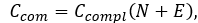where Ccompl is a coefficient of network completeness (also known as connectedness or network density), N and E is number of nodes and edges in a given network, respectively. Coefficient of network completeness can be calculated as: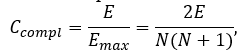where Emax is a number of edges in complete network with auto-loops.

The Ccompl is a value between 0 and 1. It shows how densely the network is populated with edges (or how close a given network is to complete network). A network which contains no edges and solely isolated nodes has a density of 0. In contrast, the density of a complete network is 1.

From two above equations the final formula for compositional complexity of network is: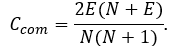Degree and neighbourhood indices (Assenov et al., 2008; Bonchev et al., 2005; Diestel, 2005; Maslov, Sneppen, 2002; Stelzl et al., 2005).

In undirected networks, the node degree ai of a node Ni is the number of edges linked to Ni: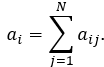A self-loop of a node is counted like one edge for the node degree. The node degree distribution gives the number of nodes with degree a for a = 0, 1, … .

The sum of all node degrees in a network defines its total adjacency A: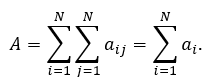The network (global or average) node degree <a> is the average of the degrees for all nodes in the network: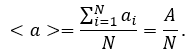The neighbourhood of a given node Ni is the set of its neighbours. The connectivity connNi of a node Ni is the number of its neighbours (or size of its neighbourhood) and it is equal to a for nodes without auto-loops or a – 1 for nodes with auto-loops. The neighbourhood connectivity NCNi of a node Ni is defined as the average connectivity of all neighbours of Ni: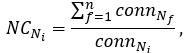where Nf is first neighbours of node Ni and n = ai (or ai – 1 for nodes with auto-loops).

The neighbourhood connectivity distribution gives the average of the neighbourhood connectivities of all nodes N with k neighbours for k = 0, 1, … .

The topological coefficient TNi of a node Ni with kn neighbours is computed as follows:Here, J(Ni, Nj) is defined for all nodes Nj that share at least one neighbour with Ni. The value J(Ni, Nj) is the number of neighbours shared between the nodes Ni and Nj, plus one if there is a direct link between Ni and Nj.

The topological coefficient is a relative measure for the extent to which a node shares neighbours with other nodes. Nodes that have one or no neighbours are assigned a topological coefficient of 0.

Clustering indices (Assenov et al., 2008; Barabási, Oltvai, 2004; Bonchev et al., 2005; Soffer et al., 2005; Watts, Strogatz, 1998).

For undirected networks, the standard definition of local clustering coefficient CNiof a node Ni is: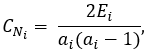where Ei is the number of edges between the first neighbours of node Ni and ai is the degree of this node.

In according to this definition, the clustering coefficient of a node Ni is the number of triangles that pass through this node, relative to the maximum number of 3-loops that could pass through the node. The clustering coefficient of a node is always a number between 0 and 1. Here, nodes with less than two neighbours are assumed to have a clustering coefficient of 0.

The global (network) clustering coefficient <c> is the average of the clustering coefficients for all nodes in the network:This is sometimes also called as transitivity of network.

The degree-correlation bias insensitive local clustering coefficient CNof a node Ni can be calculated as following: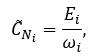where ωi is the maximum number of edges that can be drawn among the ai neighbours of a node Ni, given the degree sequence of its neighbourns {a1, …, an}(n = ai).

The global clustering coefficient <c> in that case will be equal: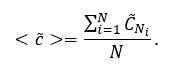Distance indices (Assenov et al., 2008; Bonchev et al., 2005).

A path in the network is a sequence of adjacent edges between two nodes without traversing any intermediate node twice. The length of a path is the number of edges forming it. There may be multiple paths connecting two given nodes. The shortest path length, also called “distance”, between two nodes Ni and Nj is denoted by d(Ni, Nj) (or simply dij). The sum of all shortest paths for a given node is node distance di: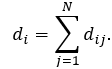The average of distances <di> for node Ni (also known as the average shortest path length or the characteristic path length) gives the expected distance between two connected nodes and can be calculated as following: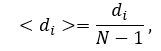or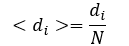for node with auto-loop.

The distance distribution gives the number of node pairs (Ni, Nj) with d(Ni, Nj) = k for k = 1, 2, … .

The sum of all shortest paths for a given network is network distance D: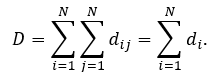The average node distance <d> is the relation of network distance to number of nodes in network: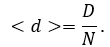The average network distance <D> (average path length or average degree of node-node separation) can be calculated by following formula:where M is number of auto-loops.

Node eccentricity ei is the maximum distance between node Ni and any of the remaining network nodes. The largest node eccentricity is termed network diameter NetD. The diameter can also be described as the largest distance between two nodes. The minimal eccentricity is termed network radius NetR. The node(s) with minimum eccentricity is defined as network centre NetC.

Centrality indices (Assenov et al., 2008; Brandes et al., 2001; Dong, Horvath, 2007; Freeman, 1977; Freeman, 1978; Newman et al., 2005; del Rio et al., 2009; Yoon et al., 2006).

The stress centrality Cs(Ni) of a node Ni is the number of shortest paths passing through Ni: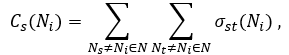where Ns and Nt are nodes in the network different from Ni and σst(Ni) is the number of shortest paths from Ns to Nt that Ni lies on.

The stress centrality value for each node Ni is normalized by dividing by the sum of centralities of the all nodes of the network: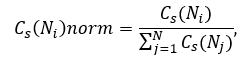where N is the total number of nodes in the network.

A node has a high stress if it is traversed by a high number of shortest paths. This parameter is defined only for networks without multiple edges. The stress centrality distribution gives the number of nodes with Cs for different values of Cs.

The betweenness centrality Cb(Ni) of a node Ni is computed as follows: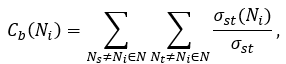where σst denotes the number of shortest paths from Ns to Nt.

Betweenness centrality is computed only for networks that do not contain multiple edges. The betweenness value for each node Ni is normalized by dividing by the number of node pairs excluding Ni: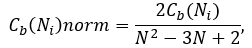where N is the total number of nodes in the connected component that Ni belongs to.

Thus, the betweenness centrality of each node is a number between 0 and 1. The betweenness centrality of a node reflects the amount of control that exerts this node over the interactions of other nodes in the network. This measure favours nodes that join communities (dense subnetworks), rather than nodes that lie inside of a community.

The closeness centrality Cc(Ni) of a node Ni is an inverse of node distance di and is computed as follows: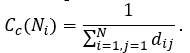Normalized value of closeness centrality for given node Ni is reciprocal value to characteristic path length and is computed as follows: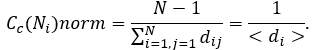The closeness centrality of each node is a number between 0 and 1. The closeness centrality of isolated nodes is equal to 0. Closeness centrality is a measure of how fast information spreads from a given node to other reachable nodes in the network.

The centralization of any network is a measure of how central its most central node is in relation to how central all the other nodes are. To calculate of network centralization, the sum of differences in centrality between the most central node in a network and all other nodes is calculated in first then this quantity is divided by the theoretically largest such sum of differences in any network of the same degree. Thus, every centrality measure can have its own centralization measure. Defined formally, if Cx(Ni) is any centrality measure of node Ni, if Cx(Nn) is the largest such measure in the network, and if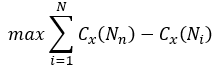is the largest sum of differences in node centrality Cx for any network of with the same number of nodes, then the centralization of the network is: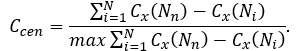Networks whose topologies resemble a star have a centralization close to 1, whereas decentralized networks are characterized by having a centralization close to 0.

For normalized values of centralities, network stress centralization can be calculated as follow: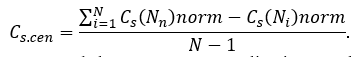Similarity, network betweenness centralization can be calculated by following way: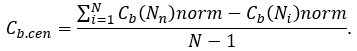As for network closeness centralization, this index can be calculated by next formula:In finally, the combined centrality score Ccs(Ni) for each gene in the network can be calculated according to the following formula:where Cx(Ni) is any centrality measure of node Ni in a given network, maxCx(Nn) and minCx(Nn) define the maximum and minimum score obtained for xth-centrality in a given network, respectively, and m refers to number of combined centralities (for instance, m = 2 for groups of 2 centralities, m = 3 for groups of 3 centralities etc.).

Combined centrality score estimates how close to the largest observed centrality measures are the centralities of the gene analysed. Thus, the higher the combined score is, the higher the individual centrality measures are.

Heterogeneity indices (Assenov et al., 2008; Dong, Horvath, 2007; Hu et al., 2008).

In undirected networks, two nodes are connected if there is a path of edges between them. Within a network, all nodes that are pairwise connected form a connected component. The number of connected components indicates the connectivity of a network – a lower number of connected components suggest a stronger connectivity.

The network heterogeneity reflects the tendency of a network to contain hub nodes. In complex scale-free networks, this parameter has significant impact on network performance, such as robustness and attack tolerance. To calculate of network heterogeneity index H, Gini coefficient-based approach is the most appropriate since it permits to quantify the heterogeneity of a network with any degree distribution and quantitatively compares the heterogeneity of networks with different types of degree distribution. In according to this approach, the H considers the difference of every two degree values in a degree sequence. Actually H is equal to on-half of the relative mean difference, i.e. the arithmetic average of the absolute values of the differences between all possible pairs of node degrees:The heterogeneity index of any given network is a number between 0 and 1 and provides a measure of the average degree inequality in a network: larger index implies higher level of heterogeneity and vice versa. For any real network H is always less than 1, and H may approach 1 only for infinite networks.

Shannon entropy.

In information theory, information entropy is a measure of unpredictability or uncertainty in a random variable. Information entropy is often called the Shannon entropy in honor of Claude E. Shannon, who in 1948 developed the first mathematical theory of entropy (Shannon, 1948). This theory links the complexity of the system, the amount of information that is needed to describe such a system, and uncertainty that arises from the transfer of information on this system through a noisy communication channel. In general, the more complex the system, the greater the amount of information needed to describe it, and the more information uncertainty that occurs when sending a message of such a system.

Consider a system S, described by the random variable X, which can take on the values x1, x2, …, xn with probabilities p1, p2, …, pn, respectively. Distribution of the values of such a variable is subject to a simple probability law:The amount of information IS needed to describe a system S in which the a priori probability of ith event is equal to 1/n is given by formula of R. Hartley (Hartley, R. V. L., 1928):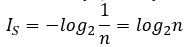Shannon entropy HS of such system is equal to the amount of information. However, Shannon entropy of a system becomes smaller than the amount of information when the probabilities of occurrence of ith events are not equal. In this case, Shannon entropy can be calculated as follow:When studying cellular networks, probability p of ith event (e.g., the likelihood that the node Ni will have a degree a) is unknown, but given only the value xi of random variable X (in the above example it is value of node degree determined in the topological analysis). In this case, the computation of the information entropy is preceded normalization of such data – the calculation of the theoretical probabilities of single events. Suppose that ai(j) is a value of the degree of the ith node in jth network (i = 1, 2, ..., N; j = 1, 2, ..., m). Then: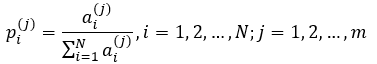At identical (equiprobable) values of degree for each node of the given network probability p of ith event is: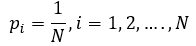Since different cellular networks may differ in a size of N, normalized Shannon entropy HSnorm should be used in comparative analysis: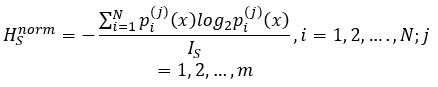### References

• Aric, A.A., Schult, D.A. and Swart, P.J. (2008). Explor-ing network structure, dynamics, and function using Net-workX. In: Varoquaux G., Vaught T., Millman J. (eds). Proceedings of the 7th Python in Science Conference (SciPy 2008). Pasadena, USA, pp. 11-15.

• Assenov, Y., Ramírez, F., Schelhorn, S.E., Lengauer, T. and Albrecht, M. (2008). Computing topological parameters of biological networks. Bioinformatics, 24, 282-284. doi: 10.1093/bioinformatics/btm554

• Barabási, A. L. and Oltvai, Z. N. (2004) Network biolo-gy: understanding the cell's functional organization. Nat. Rev. Genetic., 5, 101–113.

• Batagelj, V. and Mrvar, A. (1998). Pajek – program for large network analysis. Connections, 21, 47-57.

• Bonchev, D. and Buck, G. A. (2005) Quantitative measures of network complexity. In: Bonchev, D. and Rouvray, D. H. (eds). Complexity in chemistry, biology and ecology. Springer, New York, pp. 191–235.

• Brandes, U. (2001) A faster algorithm for betweenness centrality. // J. Math. Sociol., 25, 163–177.

• Chatr-Aryamontri, A., Breitkreutz, B.J., Heinicke, S., Boucher, L., Winter, A., Stark, C., Nixon, J., Ramage, L., Kolas, N., O’Donnell, L., Reguly, T., Breitkreutz, A., Sellam, A., Chen, D., Chang, C., Rust, J., Livstone, M., Oughtred, R., Dolinski, K. and Tyers, M. (2013). The Bi-oGRID interaction database: 2013 update. Nucleic Acids Research, 41, D816-D823. doi: 10.1093/nar/gks1158

• Csardi, G. and Nepusz, T. (2006). The igraph software package for complex network research. InterJournal, Com-plex Systems, 1695.

• Del Rio, G. et al. (2009) How to identify essential genes from molecular networks? BMC Sys. Biol., 3, 102. DOI:10.1186/1752–0509–3–102.

• Diestel, R. (2005) Graph theory. Springer-Verlag, Hei-delberg, ISBN 3–540–26182–6.

• Dong, J. and Horvath, S. (2007) Understanding network concepts in modules. BMC Sys. Biol., 1, 24. DOI:10.1186/1752–0509–1–24.

• Freeman, L. C. (1977) A set of measures of centrality based on betweenness. Sociometry, 40, 35–41.

• Hartley, R. V. L. (1928) Transmission of information. Bell Sys. Tech. J., 7, 535–563.

• Hu, H.–B. and Wang, X.–F. (2008) Unified index to quantifying heterogeneity of complex networks. Physica A, 387, 3769–3780.

• MacNeil, L.T. and Walhout, A.J.M. (2011). Gene regula-tory networks and the role of robustness and stochasticity in the control of gene expression. Genome Research, 21, 645-657. doi: 10.1101/gr.097378.109

• Margolin, A.A., Wang, K., Lim, W.K., Kustagi, M., Ne-menman, I. and Califano, A. (2006). Reverse engineering cellular networks. Nature Protocols, 1, 663-672. doi: 10.1038/nprot.2006.106

• Maslov, S. and Sneppen, K. (2002) Specificity and stability in topology of protein networks. Science, 296, 910–913.

• Newman, M. E. J. (2005) A measure of betweenness centrality based on random walks. Soc. Networks, 27, 39–54.

• Newman, M.E.J. (2010). Networks. An introduction. Ox-ford University Press, USA, 784 pp.

• Shannon, C. E. (1948) A mathematical theory of com-munication. Bell Sys. Tech. J., 27, 379–423, 623–656.

• Soffer, S. N. and Vazquez, A. (2005) Network clustering coefficient without degree-correlation biases. Phys. Rev., 71, 057101–1–057101–4.

• Stelzl, U. et al. (2005) A human protein-protein interac-tion network: a resource for annotating the proteome. Cell, 122, 957–968.

• Szklarczyk, D., Franceschini, A., Kuhn, M., Simonovic, M., Roth, A., Minguez, P., Doerks, T., Stark, M., Muller, J., Bork, P., Jensen, L.J. and von Mering, C. (2011). The STRING database in 2011: functional interaction networks of proteins, globally integrated and scored. Nucleic Acids Research, 39, D561-568. doi: 10.1093/nar/gkq973

• Watts, D. J. and Strogatz, S. H. (1998) Collective dy-namics of ‘small-world’ networks. Nature, 393, 440–442.

• Yoon, J. et al. (2006) An algorithm for modularity anal-ysis of directed and weighted biological networks based on edge-betweenness centrality. Bioinformatics, 22, 3106–3108.